### Home > CALC > Chapter Ch3 > Lesson 3.2.3 > Problem3-73

3-73.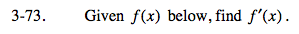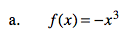Use the Power Rule.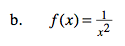f(x) can be rewritten with a negative exponent.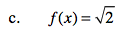f(x) can be rewritten with a fractional exponent.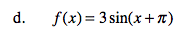You know that the derivative of y = sinx is y' = cosx. Well, f(x) is a transformation of y = sinx. So f '(x) (its slope function) will be a transformation of cosx.

The 3 represents a vertical stretch. How will that transform the derivative (slope) of y = sinx? The +π represents a horizontal shift. How will that transform the derivative (slope) of y = sinx?

The derivative will also be shifted π units to the left and stretched by a factor of 3.